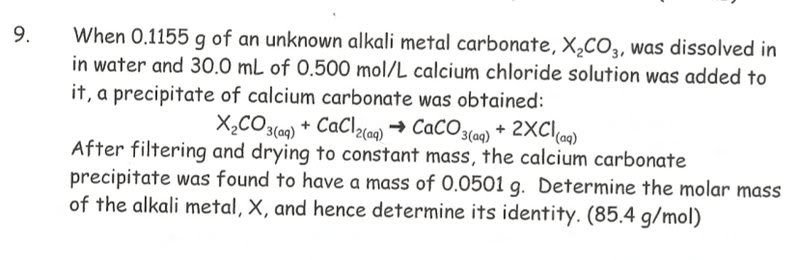September 29, 2023, 01:57:32 PM
Forum Rules: Read This Before Posting

###Topic: Identifying Unknown element After Determining Molar Mass  (Read 6177 times)

0 Members and 1 Guest are viewing this topic.

####yitriumm

• New Member
•• Posts: 7
• Mole Snacks: +0/-0##### Identifying Unknown element After Determining Molar Mass
« on: January 05, 2008, 03:51:39 PM »
The holidays are almost over and I am one question away from completing my homework. This has to be the most evil question I have ever tried to solve. Anything I do gives me the wrong answer (I know this because the teacher gave us the answer at the end of the problem, we have to show how to solve). I would appreciate it if someone explained the steps as to how so solve it.####Borek##### Re: Identifying Unknown element After Determining Molar Mass
« Reply #1 on: January 05, 2008, 04:29:37 PM »
Show how you tried.
ChemBuddy chemical calculators - stoichiometry, pH, concentration, buffer preparation, titrations.info, pH-meter.info

####Alpha-Omega

• Full Member
•• Posts: 693
• Mole Snacks: +360/-231
• Gender:• Physical Inorganic Chemist##### Re: Identifying Unknown element After Determining Molar Mass
« Reply #2 on: January 05, 2008, 04:41:35 PM »
HINT:

The question states alkali metal carbonate with formula X2CO3 and resukts in a product having the formula 2XCl....

So if you look at the periodic table and the oxidation state of the carbonate anion...and that coefficient on that product 2XClyou will be able to tell which GROUP your answer lies in....that limits what you are looking for...

So your calculation should result in an answer that corresponds to a species in that GROUP....

####Kryolith

• Full Member
••• Posts: 269
• Mole Snacks: +19/-4
• Gender:##### Re: Identifying Unknown element After Determining Molar Mass
« Reply #3 on: January 05, 2008, 04:51:39 PM »
HINT: So if you look at the periodic table and the oxidation state of the carbonate anion...and that coefficient on that product 2XClyou will be able to tell which GROUP your answer lies in....that limits what you are looking for...
The answer of the group of X is given in the text. --> "alkali metal"

Let me try to explain:

n(X2CO3):n(CaCl2):n(CaCO3)=1:1:1

n(CaCl2)=c(CaCl2)*V(CaCl2)=30 ml * 0.5 mmol/ml = 15 mmol

With m(X2CO3) and n(CaCl2), we can calculate the minimum molar mass of the carbonate that's required for a complete precipitation:

Mmin(X2CO3)=m(X2CO3)/n(X2CO3)=115.5 mg/15 mmol = 7.7 g/mol

Every possible carbonate has a molar mass greater than 7.7, so we can be sure that X2CO3 reacts completely to CaCO3. The following calculation would have worked without this step, too (but we didn't know that before).

n(CaCO3)=m(CaCO3)/M(CaCO3)=50.1 mg / 100.1 g/mol = 0.5 mmol

We know: n(X2CO3)=n(CaCO3) and M(X2CO3)=60+2x so

M(X2CO3)=m(X2CO3)/n(X2CO3) <==> 60+2x=115.5 / 0.5 mmol

==> x=M(X)=85.5 g/mol

« Last Edit: January 17, 2012, 10:46:29 AM by Arkcon »